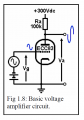# What is the purpose of resistor Ra in this simple vaccum tube voltage amplifier circuit?

#### RazRojasB

Joined Feb 24, 2017
2
Came across this in a book, there's a brief explanation of why there's said resistor in the circuit:

" ... it should be obvious that if we apply an audio signal voltage to the grid (i.e., vary the grid voltage) then current in the valve is bound to vary too. We can put this current variation to good use by putting a resistance in series with the valve so that a corresponding audio voltage is generated across the resistor, which is also called the load. "

Yet I can't quite understand the need for the resistor to be there in order to create the second amplified voltage signal, won't the amplified voltage signal be there anyway whether there's a resistor or not?

Thanks in advance for any replies.#### SLK001

Joined Nov 29, 2011
1,548
Current limiter. Without this resistor, you won't be able to generate any output, because Vout would be 300VDC.

#### RazRojasB

Joined Feb 24, 2017
2
So, to "isolate" the output where the voltage signal is being measured/taken from the 300V?

Joined Jul 18, 2013
23,620
Also the Anode load, where your output is developed across, if it were not there excessive tube current could flow, plus no amplification of signal.
Max.

#### GopherT

Joined Nov 23, 2012
8,012
1) why the resistor is there AND why the resistor is connected to 300V DC source,
Answer: the tube needs a charge from ground to anode or no current will flow. The 300 v connection is needed.

2) why is there a resistor between the 300V DC source and the tube anode?
Answer: expecting an audio signal by connecting a wire directly to a 300VDC unreasonable. The signal is essentially shorted to positive voltage rail and attenuated. Bad design, not going to work.

Google "class A" amplifier for more detail.

#### nsaspook

Joined Aug 27, 2009
8,667
You can think of (model) Ra and the tube as a variable voltage divider of the +300VDC B+ supply. Ra sets the max current to ground from the B+ if the tube resistance is close to zero as controlled by the grid voltage. If the grid voltage is adjusted and the tube resistance is high (>>Ra) Ra sets the max current to any possible load resistance from B+. For example if 1 volt of grid voltage produces an effective tube resistance of 100k the plate voltage would be 150VDC because of this voltage divider action with no extra load.

The voltage change for varying grid to plate is normally called: mu = dVp / dVg

This 'resistance' Gm = dIp / dVg change to voltage is normally measured as Transconductance#Vacuum_tubes.

#### crutschow

Joined Mar 14, 2008
27,727
You appear to have a basic misunderstanding of tube operation
A tube converts an input voltage into a plate current (the ratio between the two is the transconductance).
It doesn't directly amplify voltage into voltage.

So to get a signal voltage at the plate, the varying plate current needs to go through a resistor.
From Ohm's law the signal voltage will equal I*R when I is the plate current and R is the plate resistor (Ra).

•Ramussons and spinnaker

#### ErnieM

Joined Apr 24, 2011
8,178
Between anode (top) and cathode (bottom) is a vacuum so no current can flow. Even though the cathode is heated to provide a source of electrons to travel from cathode to anode the tube does not create current by itself. So it needs something external to drive it, here the 300 volt supply.

The grid will control how much current wil flow, but without the resistor you would not see any change for the output.

With the resistor the more current passing thru the tube the lower the output voltage goes.

#### MrChips

Joined Oct 2, 2009
24,192
The resistor is called the load. Without a load the circuit would not work.
It also establishes the possible operating I-V restraints on the circuit, called the load-line and sets the DC operating or Q-point (Q for quiescent) of the circuit.

In other words, for any applied voltage on the grid, the current and voltage on the tube must lie on the load line (the blue line shown below).

See graph below.
Blue line is the load line.
Red dot is the Q-point.In this example, the load resistor, Ra = 100kΩ

Max plate current = Va/Ra = 300V/100kΩ = 3mA

Note that the slope of the load line = -1/Ra

•spinnaker

#### EM Fields

Joined Jun 8, 2016
583
Came across this in a book, there's a brief explanation of why there's said resistor in the circuit:

" ... it should be obvious that if we apply an audio signal voltage to the grid (i.e., vary the grid voltage) then current in the valve is bound to vary too. We can put this current variation to good use by putting a resistance in series with the valve so that a corresponding audio voltage is generated across the resistor, which is also called the load. "

Yet I can't quite understand the need for the resistor to be there in order to create the second amplified voltage signal, won't the amplified voltage signal be there anyway whether there's a resistor or not?

Thanks in advance for any replies.

View attachment 121273
Regardless of the collector voltage (within reason),

the grid voltage will determine how many electrons can be released from the cloud and make the the toob look like a resistance